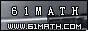Today is . WELCOME: huohai3983/44663  Home | Reg Login | All user | setHomePage | BookmarkHome | Math Test Online | Addition | Subtraction | Multiplication | Division | Time | Worksheet(JPG/PDF) | CAI |Recommendation articleArticle searchHome--maths--First Grade--Adding Three One-Digit Numbers

Adding three numbers (for example 5 + 8 + 7) involves two steps.

• Mentally add the first two numbers. (5 + 8 = 13).
• Add the other number to this sum. (13 + 7 = 20).

#### Find the Sum of the three Numbers.

 =

Viewed 2056 times CloseGreatmathsites